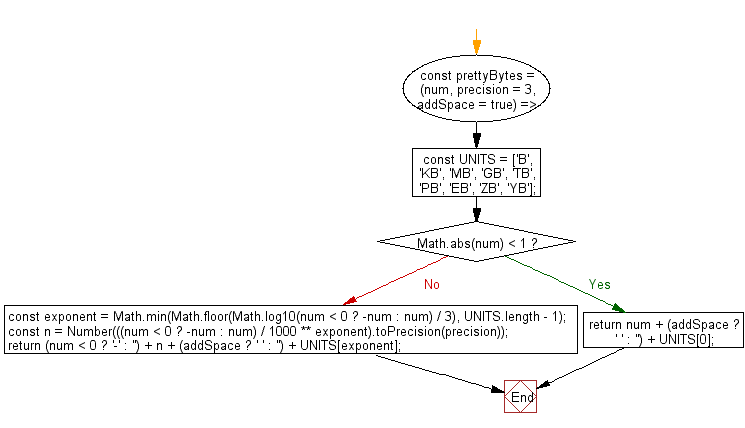# JavaScript: Convert a number in bytes to a human-readable string

## JavaScript fundamental (ES6 Syntax): Exercise-163 with Solution

Write a JavaScript program to convert a number in bytes to a human-readable string.

Note: Use an array dictionary of units to be accessed based on the exponent.

• Use an array dictionary of units to be accessed based on the exponent.
• Use Number.prototype.toPrecision() to truncate the number to a certain number of digits.
• Return the prettified string by building it up, taking into account the supplied options and whether it is negative or not.
• Omit the second argument, precision, to use a default precision of 3 digits.
• Omit the third argument, addSpace, to add space between the number and unit by default.

Sample Solution:

JavaScript Code:

``````//#Source https://bit.ly/2neWfJ2
const prettyBytes = (num, precision = 3, addSpace = true) => {
const UNITS = ['B', 'KB', 'MB', 'GB', 'TB', 'PB', 'EB', 'ZB', 'YB'];
if (Math.abs(num) < 1) return num + (addSpace ? ' ' : '') + UNITS;
const exponent = Math.min(Math.floor(Math.log10(num < 0 ? -num : num) / 3), UNITS.length - 1);
const n = Number(((num < 0 ? -num : num) / 1000 ** exponent).toPrecision(precision));
return (num < 0 ? '-' : '') + n + (addSpace ? ' ' : '') + UNITS[exponent];
};
console.log(prettyBytes(1000));
console.log(prettyBytes(-27145424323.5821, 5));
console.log(prettyBytes(123456789, 3, false));
```
```

Sample Output:

```1 KB
-27.145 GB
123MB
```

Pictorial Presentation:Flowchart:Live Demo:

See the Pen javascript-basic-exercise-163-1 by w3resource (@w3resource) on CodePen.

Improve this sample solution and post your code through Disqus

What is the difficulty level of this exercise?

Test your Programming skills with w3resource's quiz.

﻿